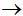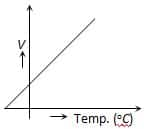Engineering Entrance Sample Papers

WBJEEM Chemistry Entrance Exam Practice Question PaperWest Bengal Joint Entrance Examinations Board (WBJEEM) chemistry 50 questions and answers.

Ques. The ratio of the amount of H2S needed to precipitate all the metal ions from 100 c.c. of 1 M AgNO3 and 100 c.c. of 1 M CuSO4 will be
(a) 1 : 1
(b) 2 : 1
(c) 2 : 1
(d) Indefinite
Ans. (c)

Ques. When 10 gm of Na2SO4, xH2O is heated to dryness 5.035 gm water vapour is produced, therefore the value of x is
(a) 10
(b) 7
(c) 6
(d) 8
Ans. (d)

Ques. HI was heated in a sealed tube at 400°C till the equilibrium was stablished. HI was found to be 22% decomposed. The equilibrium constant for dissociation HI D ½ H2 + ½ I2 is
(a) 0.282
(b) 0.0796
(c) 0.0199
(d) 1.99
Ans. (c)

Ques. One mole of mixture of N2, NO2 and N2O4 has a mean molar mass of 55.4. On heating to a temperature at which all the N2O4 may be presumed to have dissociated : N2O4 D 2NO2, the mean molar mass tends to the lower value of 39.6. What is the mole ratio of N2 : NO2 : N2O4 in the original mixture
(a) 0.5 : 0.1 : 0.4
(b) 0.6 : 0.1 : 0.3
(c) 0.5 : 0.2 : 0.3
(d) 0.6 : 0.2 : 0.2
Ans. (a)

Ques. X is a 100 ml solution of HNO3 whose dilution is 1000. Y is the second 100 ml of solution of HNO3 whose concentration is C. The pH becomes 1 on mixing X and Y in a beaker, then the value of C will be
(a) 0.199 N
(b) 0.10 N
(c) 0.30 N
(d) 0.50 N
Ans. (a)

Ques. What is the pH value of 1 N CH3COONH4
(a) ½ pKw
(b) pKw
(c) 2 pKw
(d) 12 pKw
Ans. (a)

Ques. A cylinder of compressed gas bears no label is supposed to contain either ethylene (C2H4) or propylene (C3H6). Combustion of the gaseous sample shows that 12mL of gas required 54 mL of oxygen for complete combustion. This indicates that the gas is
(a) Only ethylene
(b) Only propylene
(c)  mixture of two gases
(d) Some unknown mixture of two gases
Ans. (b)

Ques. At the top of the mountain the thermometer reads 0°C and the barometer reads 710 mm Hg. At the bottom of the mountain the temperature is 30°C and pressure is 760 mm Hg. Density of air at the top with that at the bottom is
(a) 1 : 1
(b) 1.04 : 1
(c) 1 : 1.04
(d) 1.15
Ans. (b)

Related: Trigonometry Question Bank

Ques. A solution of 500 ml of 0.2 M KOH and 500 ml of 0.2M HCl is mixed and stirred; the rise in temperature is T1. The experiment is repeated using 250 ml each of solution, the temperature raised is T2. Which of the following is true
(a) T1 = T2
(b) T1 = 2T2
(c) T1 = 4T2
(d) T2 = 9T1
Ans. (a)

Ques. The indicator used in the titration of KMnO4 against FeSO4 is
(a) Phenolphthalein
(b) K3Fe(CN)6
(c) None
(d) Methyl orange
Ans. (c)

Ques. 2 gm of a metal when treated with air gave 2.8 gm of its oxide. The molecular formula of its chloride is MCl2. The atomic weight of the metal is
(a) 20
(b) 40
(c) 60
(d) 80
Ans. (b)

Ques. Oxidation number of phosphorus in Ba(H2PO2)2 is
(a) +3
(b) +2
(c) +1
(d) –1
Ans. (c)

Ques. How many primary, secondary, tertiary and quaternary carbons are present in the following hydrocarbon CH3 – CH(CH3) – C(CH3)2 – CH2 – CH(CH3) – CH2 – CH3

 Primary Secondary Tertiary Quaternary (a) 6 2 2 1 (b) 2 6 3 0 (c) 2 4 3 2 (d) 2 2 4 3

Ans. (a)

Ques. Stability number of neutrons is present in which of the following elements
(a) 13Al27
(b) 83Bi209
(c) 92U238
(d) 26Fe56
Ans. (b)

Ques. Wavelength of gamma rays is
(a) 10–7 m
(b) 10–10 m
(c) 10–8 m
(d) 10–12 m
Ans. (d)

Ques. Which of the following statements is false
(a) Catalyst starts the reaction
(b) Catalyst remains unchanged in amount and constitution after the reaction
(c) Catalyst does not shift the equilibrium in a reversible reaction
(d) None of these
Ans. (a)

Ques. The catalyst used in the manufacture of ether from alcohol is
(a) Al2O3
(b) CrO3
(c) MnO
(d) None of these
Ans. (a)

Ques. In the Fischer-Tropsch synthesis of petrol….. and ….. are used as the raw materials
(a) H2 ; CO
(b) CH4 ; H2
(c) CH4 ; CH3OH
(d) CH3 OH ; CO
Ans. (a)

Ques. According to Hardy-Schulze low, in order to coagulate a certain amount of a colloid, the amount of electrolyt used depends on
(a) Opposite charge on ion
(b) Size of ion
(c) Nature of ion
(d) Shape of ion
Ans. (a)

Ques. Oxidation number of S in H2S2O7 is
(a) +4
(b) –6
(c) –5
(d) +6
Ans. (d)

Ques. A gas decolourises bromine water and on reacting with hydrogen bromide forms bromoethene and ethylidene bromide the gas is
(a) Propane
(b) Propene
(c) Ethene
(d) Ethyne
Ans. (d)

Ques. Size of a colloidal particle is
(a) 10 – 1000 Å
(b) 200-100 Å
(c) 20–50 Å
(d) 1–20 Å
Ans. (a)

Ques. Monomer of neoprene is
(a) Isoprene
(b) Chloroprene
(c) Vinyl chloride
(d) Vinyl acetylene
Ans. (b)

Ques. Acelylene on polymerization gives
(a) Vinyl acetylene
(b) Cyclooctatetraene
(c) Benzene
(d) All of these
Ans. (d)

Ques. Which of the following sols is positively charged?
(a) As2O3
(b) Fe(OH)3
(c) Au
(d) Starch
Ans. (b)

Ques. Which of the following compounds does not form a carbonyl compound on hydrolysis?
(a) (CH3)3CCHCl2
(b) (CH3)3CCCl3
(c) (CH3)2CCl2
(d) (CH3)2CHCHCl2
Ans. (b)

Ques. Which of the following types of reactions is not exhibited by diethyl ether
(a) Salt formation
(b) Neucleophilic substitution
(d) Electrophilic substitution
Ans. (d)

Ques. Which of the following products is formed on the reaction of diethyl ether with chlorine in the presence of direct sunlight
(a) CCl3 – CH2 – O – CH2 – CCl3
(b) ClCH2 – CH2 – O – CH2 –CH2Cl
(c) CCl3 – C(Cl2) – O – C(Cl2) – CCl3
(d) (CH3 – CH – Cl)2O
Ans. (c)

Ques. Which of the following acids does not form phenol when its sodium salt is dry distilled with soda lime?
(a) p-Hydroxybenzoic acid
(b) Salicylic acid
(c) m-Hydroxybenzoic acid
(d) Aspirin
Ans. (d)

Ques. The values of dissociation constants of some acids (at 25oC) are as follows. Indicate which is the strongest acid in water
(a) 1.4 x 10–2
(b) 1.6 x 10–4
(c) 4.4 x 10–10
(d) 4.3 x 10–7
Ans. (a)

Ques. The 2N aqueous solution of H2SO4 contains
(a) 49 gm of H2SO4 per litre of solution
(b) 4.9 gm of H2SO4 per litre of solution
(c) 98 gm of H2SO4 per litre of solution
(d) 9.8 gm of H2SO4 per litre of solution
Ans. (c)

Ques: Which one of the following compounds when heated with KOH and a primary amine gives carbylamine test
(a) CHCl3
(b) CH3Cl
(c) CH3OH
(d) CH3CN
Ans: (a)

Ques. The equivalent weight of phosphoric acid (H3PO4) in the reaction, NaOH + H3PO4NaH2PO4 + H2O is
(a) 25
(b) 49
(c) 59
(d) 98
Ans. (d)

Ques. From the given sets of quantum numbers the one that is inconsistent with the theory is
(a) n = 3; l = 2; m = –3; s = +1/2
(b) n = 4; l = 3; m = 3; s = +1/2
(c) n = 2; l = 1; m = 0; s = –1/2
(d) n = 4; l = 3; m = 2; s = +1/2
Ans. (a)

Ques. In which of the following reactions, there is no change in the valency
(a) 4KClO33KClO4 + KCl
(b) SO2 + 2H2S2H2O + 3S
(c) BaO2 + H­2SO4BaSO4 + H2O2
(d) 2BaO + O22BaO2
Ans. (c)

Ques. The following graph illustrates(a) Dalton’s law
(b) Charle’s law
(c) Boyle’s law
(d) Gay-Lussac’s law
Ans. (b)

Ques. One mole of SO3 was placed in a litre reaction vessel at a certain temperature. The following equilibrium was established  2SO3 ⇌ 2 SO2 + O2. At equilibrium 0.6 moles of SO2 were formed. The equilibrium constant of the reaction will be
(a) 0.36
(b) 0.45
(c) 0.54
(d) 0.675
Ans. (d)

Ques. The ability of an ion to bring about coagulation of a given colloid depends upon
(a) Its size
(b) The magnitude of its charge only
(c) The sign of its charge
(d) Both the magnitude and the sign of its charge
Ans. (d)

Ques. Aluminium is a self–preserving metal, because
(a) It is not tarnished by air
(b) A thin film of basic carbonate on its surface
(c) A non-porous layer of oxide is formed on its surface
(d) It is not affected by salt water
Ans. (c)

Ques. There is a negative charge on As2S3 sol. Which of the following salts maximum capacity of precipitating it
(a) AlCl3
(b) Na3PO4
(c) CaCl2
(d) K2SO4
Ans. (a)

Ques. Coagulation power in which of the following is maximum
(a) Pb+2
(b) Pb+4
(c) Sr+2
(d) Na+
Ans. (b)

Ques. Which of the following statements is false
(a) Physical adsorption occurs due to van der Waals forces
(b) Chemical adsorption decreases at high temperature and low pressure
(d) All the above statements are true
Ans. (d)

Ques. P4 + 3NaOH + 3H2O – – A + 3NaH2PO2 here, ‘A‘ is
(a) NH3
(b) PH3
(c) H3PO4
(d) H3PO3
Ans. (b)

Ques. The correct order of ionic radii of Y3–, La3+, Eu3+ and Lu3+ is
(a) La3+ < Eu3+ < Lu3+ < Y3+
(b) Y3– < La3+ < Eu3+ < Lu3+
(c) Lu3+ < Y3+ < Eu3+ < La3+
(d) Lu3+ < Eu3+ < La3+ < Y3+
(Atomic No. Y = 39, La = 57, Eu = 63, Lu = 71)
Ans. (c)

Ques. Credit for the ring structure of benzene goes to
(a) Wholer
(c) Kekule
(d) Baeyer
Ans. (c)

Ques. Gold number of which of the following is minimum
(a) Gelatine
(b) Starch
(c) Gum
(d) Equal for all of the above
Ans. (a)

Ques. Concentration of Ca+2 is 40 mg/litre for hard water, then how many mg of Na2CO3 will be required to prepare soft water from 5 liters of hard water
(a) 530
(b) 5.3
(c) 1.06
(d) 10.6
Ans. (a)

Ques. Dimethyl ether and ethyl alcohol are
(a) Metamers
(b) Homologues
(c) Functional isomers
(d) Position isomers
Ans. (c)

Ques. When a beam of light is passed through a colloidal solution, then this beam of light?
(a) Gets diffracted
(b) Passes unchanged
(c) Shows a rainbow
(d) Gets dispersed
Ans. (d)

Ques. Nature of charge on the particles can be determined, by which of the following properties of a colloidal systems
(a) Electrophoriesis
(b) Dialysis
(c) Absorption
(d) Ultamicrofiltration
Ans. (a)

Ques. Which of the following is the reasons for the sky generally appearing blue?
(a) Deviation effect
(b) Conduction
(c) Reflection
(d) Dispersion
Ans. (d)